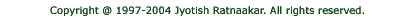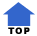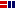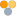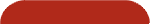About Vedic NumerologyNeed of suitable name for new born babyLucky number, alphabet suitability and phonetically correct nameOrder reportCase studiesReport Samples :--Naming your new born babyChanging your own nameNaming the new companyKnow suitable syllables for youPractical uses of "Best number" in daily lifeStock-market numerologyCorporate numerologyMaster table of Chaldean numerologyF.A.Qs.TestimonialsAbout Shyam S KansalPrivacy policyDisclaimerSite-mapAbout usContactHow to calculate Primary root-numbers?

The Chaldean System of numerology has its roots in Ancient Babylon. This system is related to the Vedic System of India and the Kabbalah System of the Hebrew mystics. The underlying premise of Chaldean numerology is that every letter has a unique vibration. Letters are assigned numeric values, from 1 to 8, based on the vibrational value. The number 9 (nine) is considered 'sacred' and is not assigned to any letter. Numeric equivalents to Roman alphabets are same in Chaldean System of numerology and Vedic numerology.

To view the table of numeric equivalents to Roman alphabets / Chaldean Conversion Table (numbers assigned to various alphabets) - Click here.

To interpret a name numerically, assign a number to each alphabet based on the Chaldean numerology system, and add these numbers together. Keep adding until you get a single digit.

If the figure thus arrived is a single digit (from 1 to 9), then the primary root number is the figure itself. (For example, if the figure arrived after a sum total of four alphabets is 1+3+2+1 = 7).

Else, if the figure thus arrived is not a single digit figure (10 or greater), then, the thus obtained two or more digits are numerically further added together till they form a single digit. This process should be repeated until you get a single digit. This single digit will then become the primary root number. For example, if the initial total of Chaldean values comes as 84. Then, add 8 & 4 together. 8 + 4 = 12. This is a two digit figure, thus, again add (1 + 2) together and accept the resultant single digit "3" as primary root number.
 See a typical sample of primary root number calculation: ```*---- VELUPILLAI PRABHAKARAN VELUPILLAI 6536813311 = 37 = 1 PRABHAKARAN 82125121215 = 30 = 3 [ 1 + 3 = 4 ] The primary root number of the name is "FOUR".```